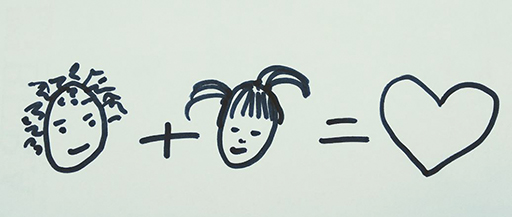Teaching mathematics

Start this free course now. Just create an account and sign in. Enrol and complete the course for a free statement of participation or digital badge if available.

Free course

# 3 The equals signFigure 7 Friends = love

One of the strong findings of early algebra research is that learners in the middle years need to change their perception of the equals sign. This sign is recognised from an early age (Figure 7), and it is usually understood as standing for an instruction such as ‘makes’ or ‘work it out now’. This is called the operational understanding of the equals sign and it works well for number problems. Text books, worksheets and even national examinations use the equals sign in this way:

To make progress in algebraic thinking, learners need to develop a new relational understanding of the equals sign. In algebra, the equals sign only makes sense when it is used as a way of comparing two expressions and saying that they have the same value as each other.

All of the following equations can be understood as statements that express a relationship between two quantities:

Two other relational symbols are the inequality signs < and >. Tasks that require learners to decide which of these symbols to use can develop a relational understanding of the equals sign.

## Activity 13 Relationships between expressions

Timing: Allow 5 minutes

Which is bigger: 3ab or 2a + b? Choose values of a and b and decide. Record your results using the signs <, > and =.

### Discussion

You could write:

If we choose then and , so .

If then and so .

Or then and

## Activity 14 Reflect

Timing: Allow 5 minutes

You might have noticed the different ways in which the three responses to the previous question were written.

Does this ensure that they have practice in selecting and writing the correct signs, in comparing two expressions?

 a b 3a – b 2a + b Write your inequality or equation here 1 2 1 4 3a – b < 2a + b 10 3 6 3

### Discussion

Using the equals or inequality signs between numbers or symbolic expressions is correct mathematics. Learners should not write =, < or > on its own.

Using a table is often helpful for keeping track of several calculations.

 a b 3a – b 2a + b Write your inequality or equation here 1 2 1 4 3a – b < 2a + b 10 3 27 23 3a – b > 2a + b 6 3 15 15 3a – b = 2a + b
TM_1